# Catch up on the latest AI articles3 main points
✔️ A new Grad-CAM based method using Integrated Gradients
✔️ Satisfies the sensitivity theorem, which is a problem of gradient-based methods, because it uses the integration of gradients
✔️ Improved performance in terms of "understandability" and "fidelity" compared to Grad-CAM and Grad-CAM++.

written by Sam SattarzadehMahesh SudhakarKonstantinos N. PlataniotisJongseong JangYeonjeong JeongHyunwoo Kim
(Submitted on 15 Feb 2021)
Comments: Accepted by ICASSP 2021

Subjects: Computer Vision and Pattern Recognition (cs.CV)code：## First of all

Convolutional neural networks (CNNs) have powerful capabilities in feature representation and image recognition, but lack explainability due to their complexity, limiting users' confidence in their decisions. Explainable AI (XAI) is a field that attempts to gain trust in AI models by revealing the basis for the model's estimates.

In particular, the most famous of the XAI methods that explain CNN predictions are visualization methods (attribution methods). They are one of the "post-hoc" algorithms that interpret the behavior for a trained model.

One group of visual XAI approaches is based on the Class Activation Mapping (CAM) method. These methods include Grad-CAM and Grad-CAM++, which are very versatile and widely used.

However, because these methods use gradients, they can potentially underestimate the sensitivity of the model output to features in the image (a violation of the sensitivity axiom). To address this issue, this research solves the problem by incorporating a method called Integrated Gradients into Grad-CAM.

## Gradient-based visualization methods and sensitivity theorems

In gradient-based visualization methods, it is desirable to satisfy the axiom of sensitivity. What is the axiom of sensitivity?

For all inputs and baselines where one feature is different and the estimates are different, the different features should be given a non-zero attribution (gradient)

is.

To illustrate the axiom of sensitivity, I will explain a simple example. Let us consider $f(x)=1-\rm{ReLU}(1-x)$. Let $f(x)$ be a simple network consisting only of ReLU and $x$ is the input. From $x=0$ to $x=1$ the slope is always 1.

However, when $x>1$, the slope will always be zero. Creating heatmaps using gradient violates the axiom of sensitivity because it may create the same heatmap even if the inputs are different. Grad-CAM also violates this sensitivity axiom because it is a gradient-based method.

## Proposed method (Integrated Gradients)

The proposed method satisfies the sensitivity theorem by gradually varying the input image according to the method of Integrated Gradients in computing the gradient of the output with respect to the Grad-CAM feature map.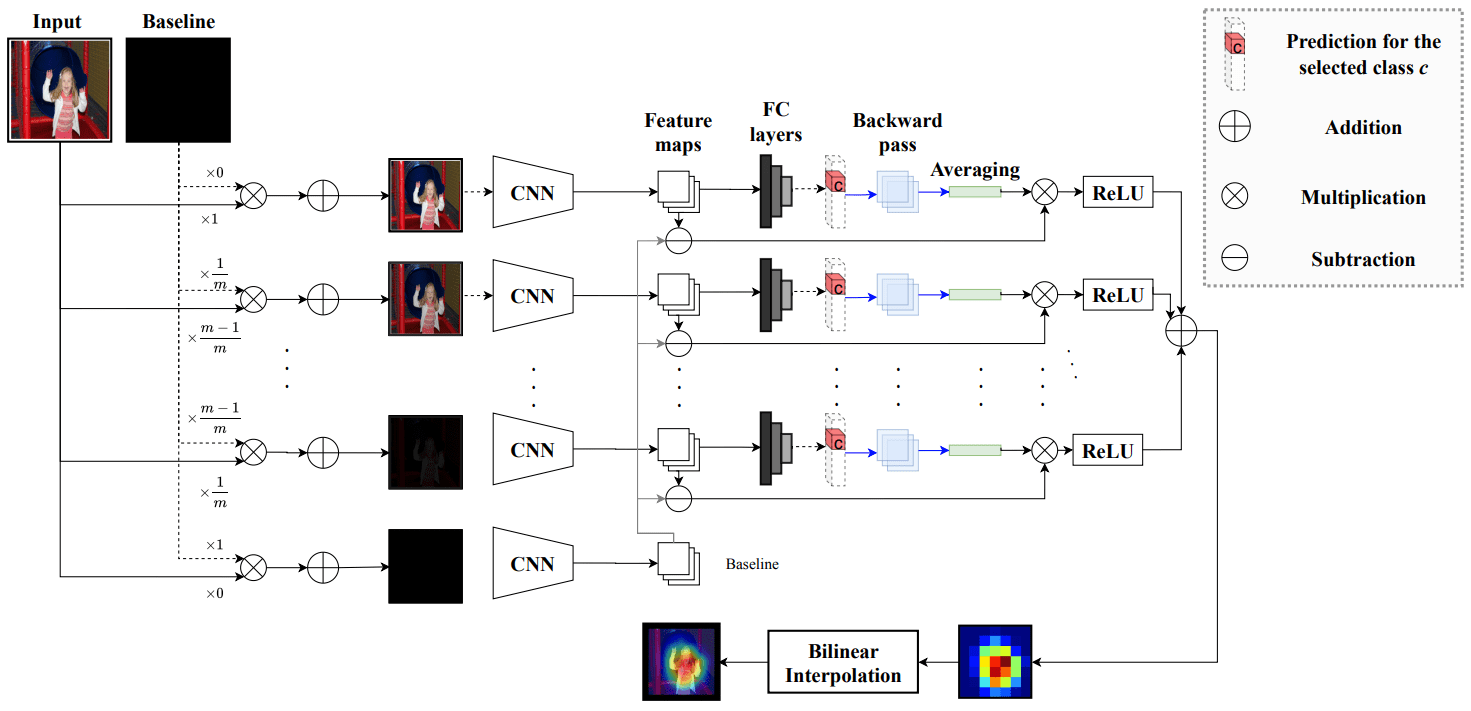The baseline is a black image. The input image produces intermediate images that gradually approach the baseline according to the number of steps $m$. Those images are input to the trained model to compute the gradient with respect to a particular feature map and output. Usually, the feature map is chosen to be the final convolutional layer.

The calculated gradient is used for scaling purposes as the difference between the gradient and the gradient obtained from the input baseline image.

Finally, Integrated Gradients can be calculated by calculating Grad-CAM for each image and taking the average.

The formula for Integrated Gradients is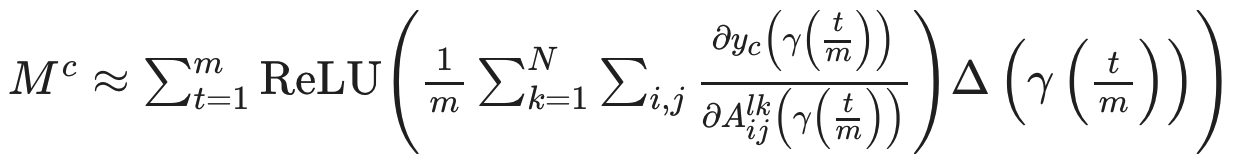can be represented by where $m$ is the number of steps that control the number of intermediate images generated, $y^c$ is an estimate of the class $c$, and $A_{i j}^{l k}$ is a feature map $k$ of size $i \times j$ of layer $l$.

$\gamma$ denotes the intermediate image, where the input image changes to the baseline linearly according to $t$. $\Delta$ refers to the difference between the gradients calculated for the intermediate image and the baseline.

## Experimental results

In this paper, we use VGG16 and ResNet-50 trained on the PASCAL VOC2007 dataset for visualization, with top-1 accuracy of 87.18 % and 87.96 %, respectively.

The results of the experiment are summarized in the following table.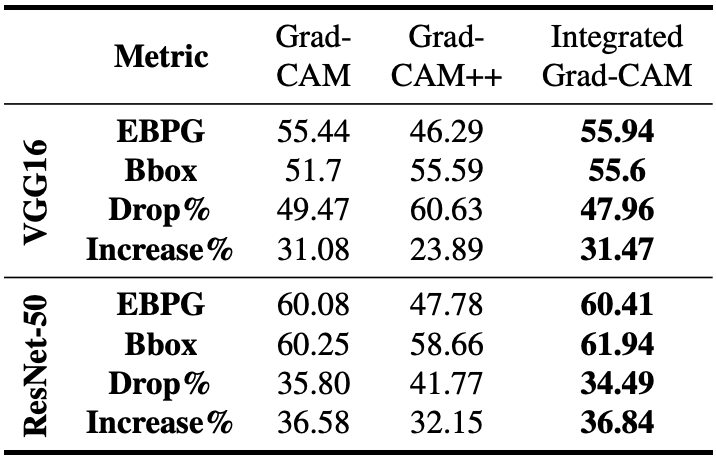The Energy-base pointing game and the Bounding box, which evaluate the localizability (understandability) of visualization methods, show better results than Grad-CAM and Grad-CAM++.

Similarly, the Drop\Increase rate, which indicates the fidelity of the explanation, also shows better results than the conventional method.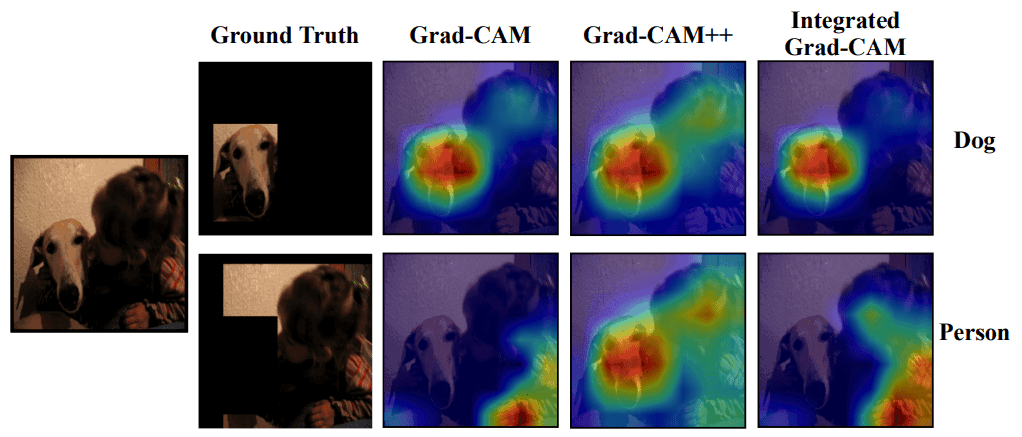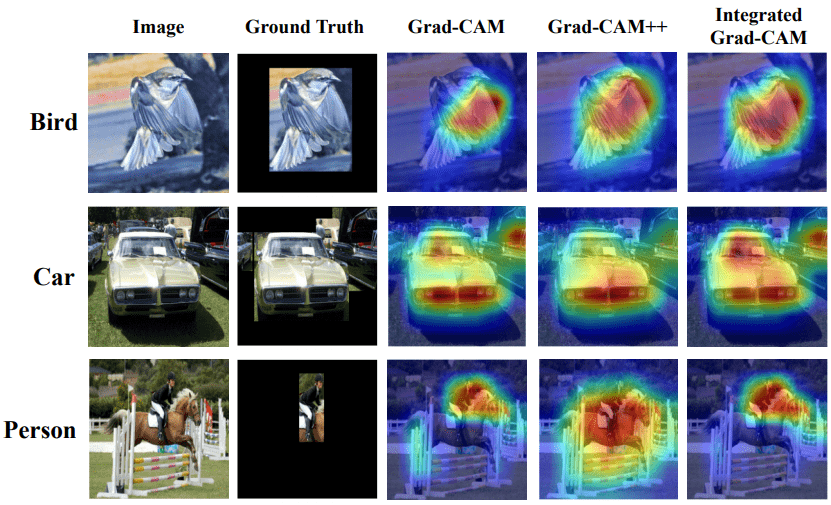An example of a visualized image shows that the proposed method captures the more important features of the true value. We randomly extracted 100 images from the PASCALVOC 2007 test set used for the ResNet-50 model and calculated the computation time per image on a 16GB P100-PCle GPU to calculate the computation time per image.

As a result, the average computation time for Grad-CAM and Grad-CAM++ is 11.3 ms, while the proposed method requires 54.8 ms on average.

## Summary

In this paper, we proposed a method to address the tendency of gradient-based visualization methods, such as Grad-CAM, to underestimate the importance of features by computing the integral of the gradient between the input image and the baseline. Compared with Grad-CAM and Grad-CAM++, the proposed method shows better results in terms of "understandability of explanation" and "fidelity of explanation".

I felt that the simple but reliable method of introducing Integrated Gradients accurately addressed the problems of gradient-based methods. In addition, since the evaluation of EBPG and Bbox was improved by simply emphasizing the features in the bounding box, there was no significant difference between them and the conventional methods, but the Drop\Increase rate, which evaluates the performance of the visualization method to show the basis of model estimation more directly, showed good results. Therefore, I think that this method has the potential to become the next standard method to replace Grad-CAM.

Since visualization is basically not a field where computational speed is required, we believe that the increase in computational time is not a problem. However, since we have not compared our method with Score-CAM and Ablation CAM, which have appeared recently, we were curious about what kind of results we would get when comparing them.

If you have any suggestions for improvement of the content of the article,
please contact the AI-SCHOLAR editorial team through the contact form.